CBSE Class 10 Sample Paper for 2019 Boards

Class 10
Solutions of Sample Papers for Class 10 Boards

Question 26

Draw a ∆ABC with sides 6 cm, 8 cm and 9 cm and then construct a triangle similar to ∆ABC whose sides are 3/5 of the corresponding sides of ∆ABC.

This is a construction Question from the Maths Sample Paper .

Every year, 1 construction question is asked. This time, we have to just construct, and not justify.

Other times, you might be asked to justify the construction as well. Which you can look at in the Constructions - Class 10 Chapter.

The solution to this question is below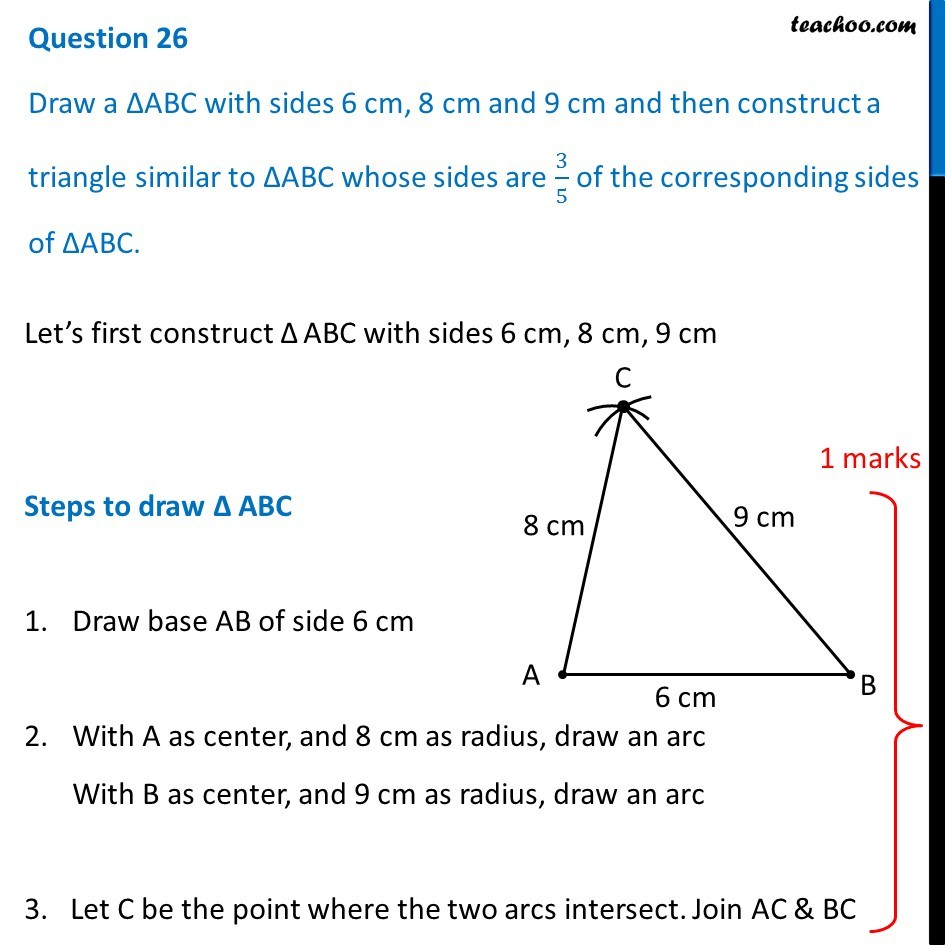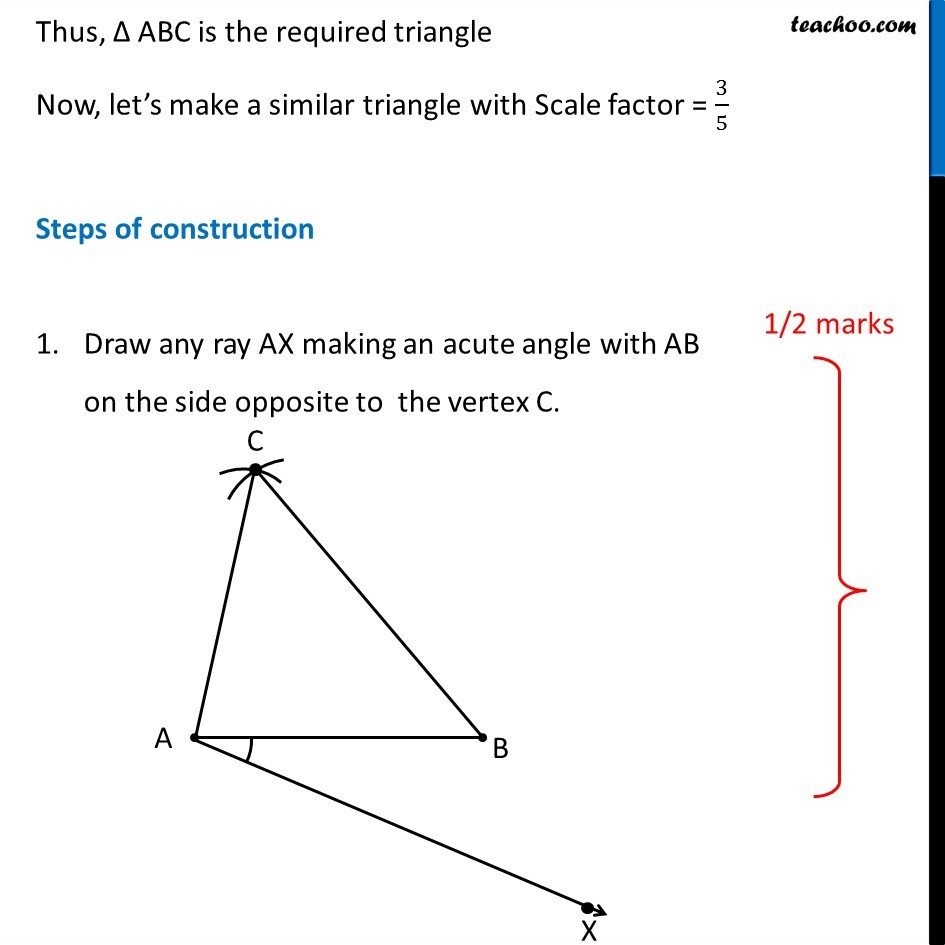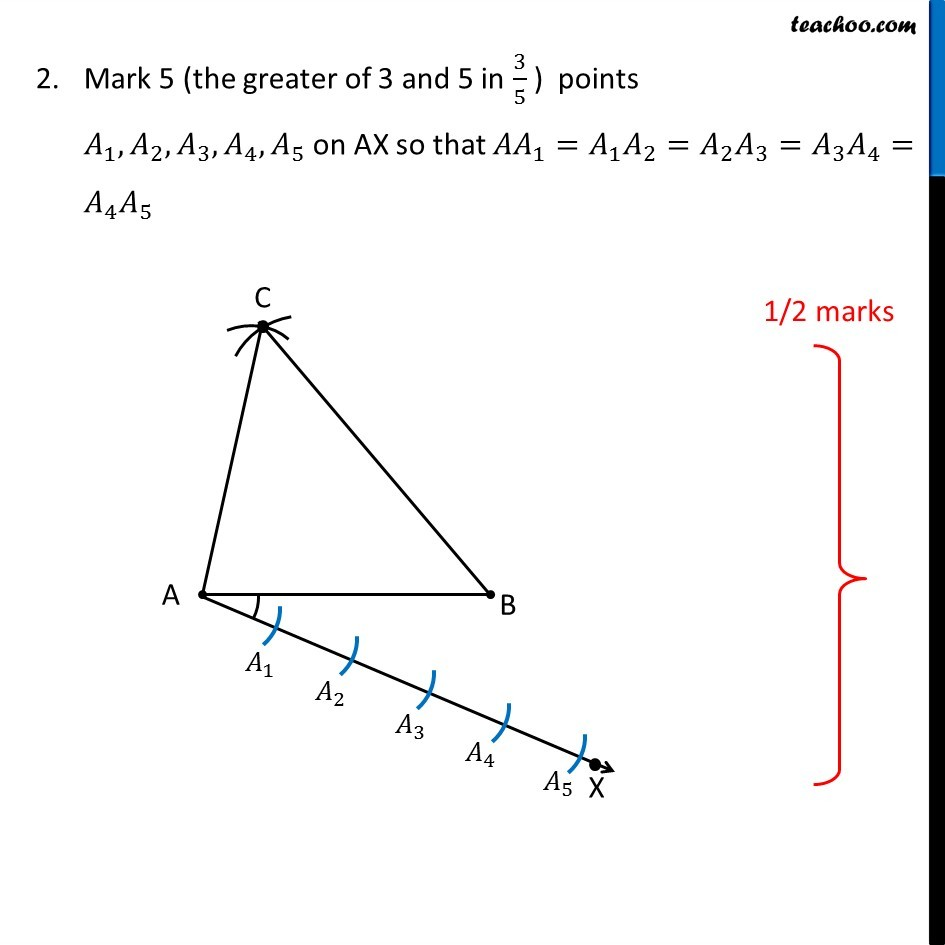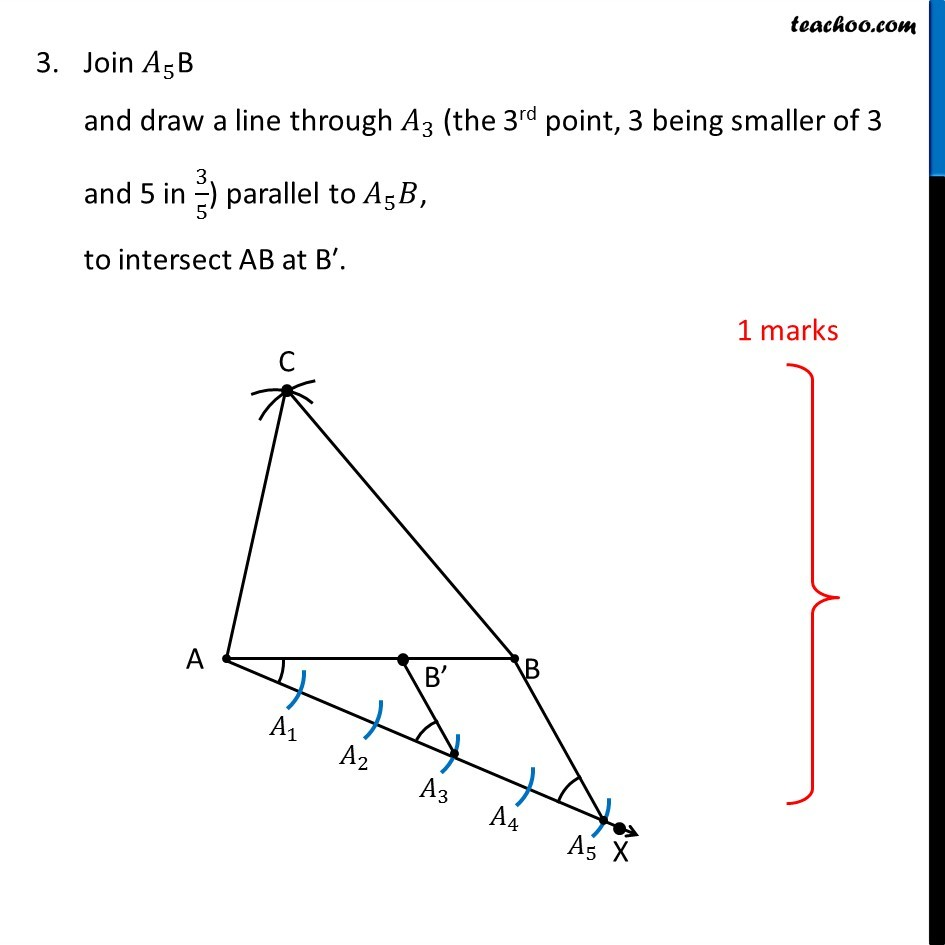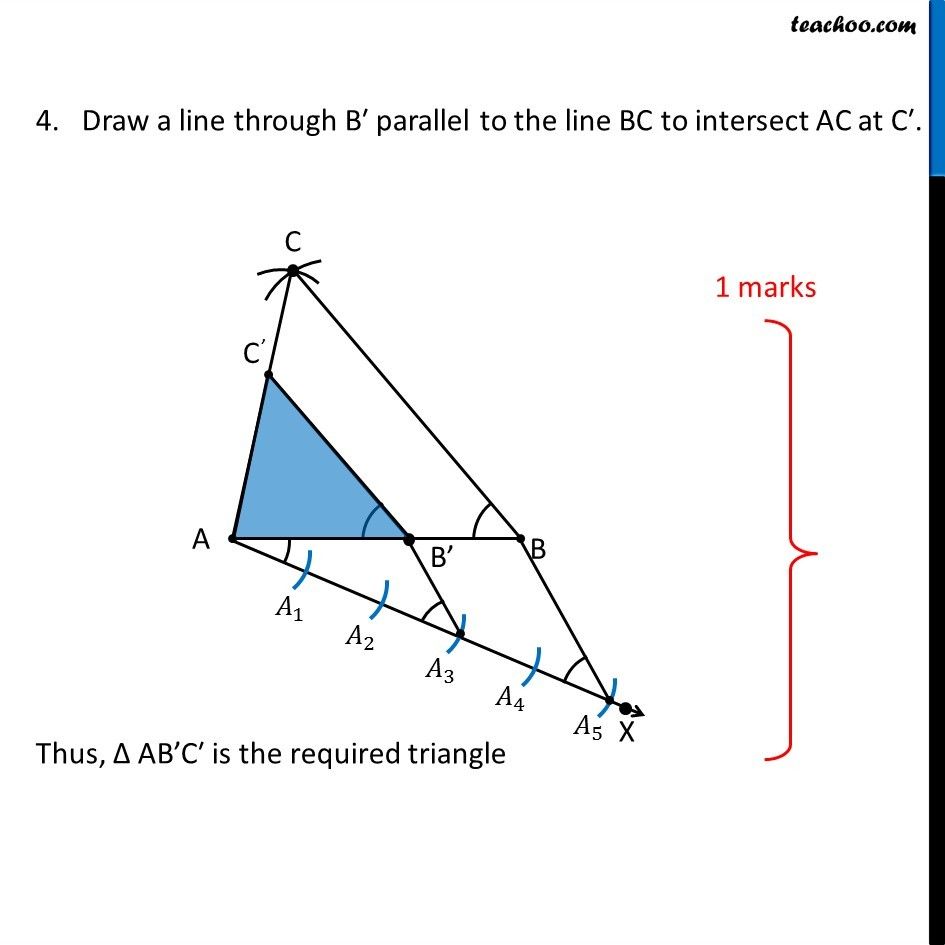Learn in your speed, with individual attention - Teachoo Maths 1-on-1 Class

### Transcript

Question 26 Draw a ∆ABC with sides 6 cm, 8 cm and 9 cm and then construct a triangle similar to ∆ABC whose sides are 3/5 of the corresponding sides of ∆ABC. Let’s first construct Δ ABC with sides 6 cm, 8 cm, 9 cm Steps to draw Δ ABC Draw base AB of side 6 cm With A as center, and 8 cm as radius, draw an arc With B as center, and 9 cm as radius, draw an arc 3. Let C be the point where the two arcs intersect. Join AC & BC Thus, Δ ABC is the required triangle Now, let’s make a similar triangle with Scale factor = 3/5 Steps of construction Draw any ray AX making an acute angle with AB on the side opposite to the vertex C. Mark 5 (the greater of 3 and 5 in 3/5 ) points 𝐴_1, 𝐴_2, 𝐴_3, 𝐴_4, 𝐴_5 on AX so that 〖𝐴𝐴〗_1=𝐴_1 𝐴_2=𝐴_2 𝐴_3=𝐴_3 𝐴_4=𝐴_4 𝐴_5 Join 𝐴_5B and draw a line through 𝐴_3 (the 3rd point, 3 being smaller of 3 and 5 in 3/5) parallel to 𝐴_5 𝐵, to intersect AB at B′. 4. Draw a line through B′ parallel to the line BC to intersect AC at C′. Thus, Δ AB’C′ is the required triangle